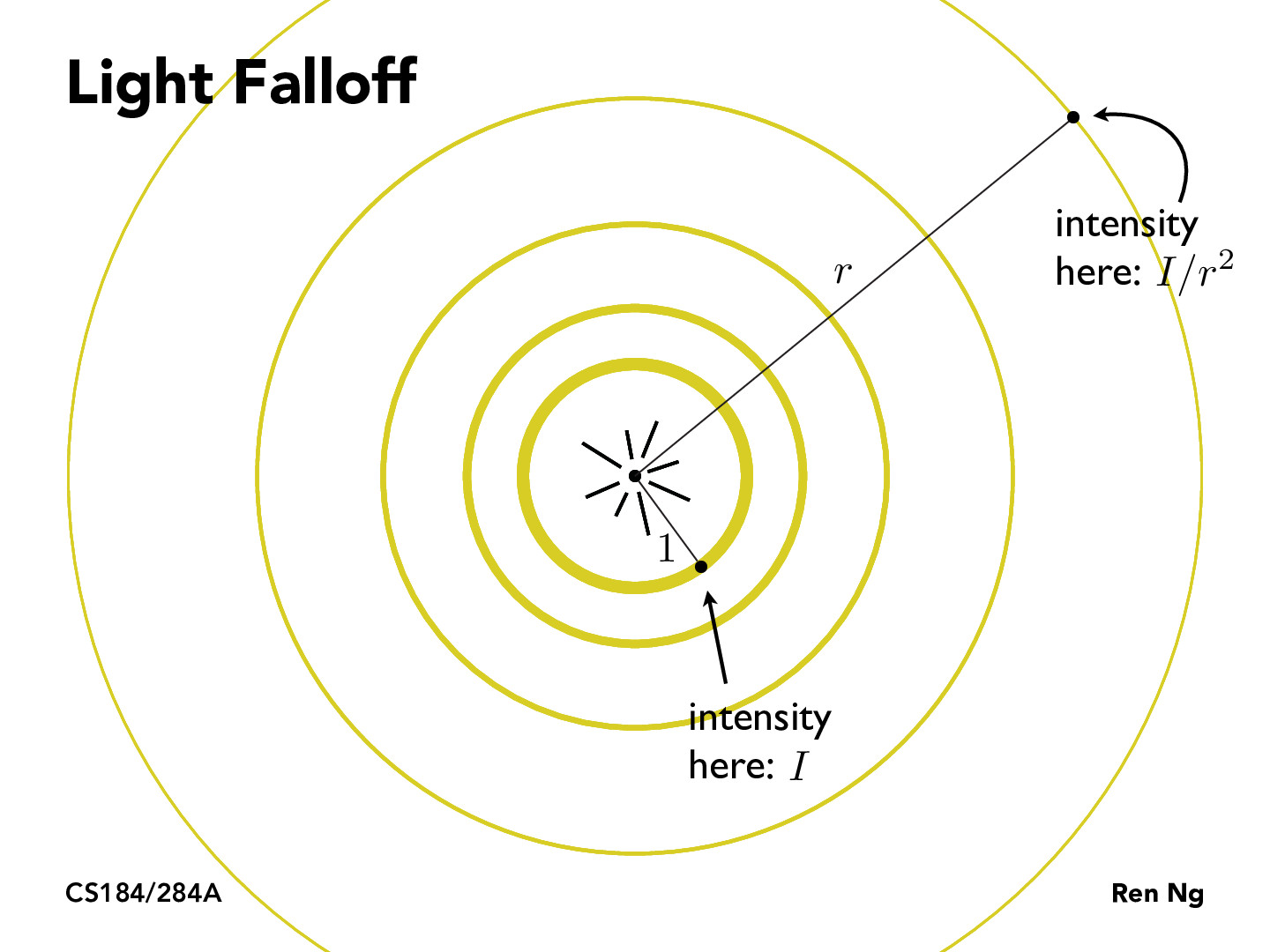You are viewing the course site for a past offering of this course. The current offering may be found here.Lecture 6: The Rasterization Pipeline (21)ellenluo

This connects to the intensity equation often used for sound in physics. The equation is I=P/A (I=intensity, P=pressure, A=area). In this case, since we're looking at a circle of radius r, I is inversely proportional to r^2 (since the area of the circle is pi * r^2). This is where the relative intensity formula on this slide comes from!frankieeder

Does this mean that our intensity is always defined at 1 unit away from the source (r=1)? Doesn't this become arbitrary if we change units or scale? By this model, couldn't we get theoretically infinite intensity as r -> 0, or do most graphics applications that our light is the unit sphere?lhagaman

You would get theoretically infinite intensity as r->0. The resolution is that light never comes from a single point in the real world (aside from small discrete numbers of photons being produced in individual interactions with other particles, which don't define an intensity since we can't properly average the rate over time), the object producing the light has some nonzero volume.AronisGod

Maybe not intensity, but certainly you can count the number of quanta and scale it by a scattering rate. If we are talking atomic emission, we can construct a wave-packet using coefficients obtained by solving Schrodinger's equation describing transitions between bound->continuum excited states (where the transition is driven by oscillating or strong static electromagnetic fields). These coefficients are thrown at a Fourier series construction of the packet. The rate can be estimated if we know more about the laser power spectrum, the relative polarizations of the electrons/laser, and the linewidth of the orbitals we are coupling to. Nonetheless, the intensity will not approach infinity because you cannot localize any excitation or particle so infinitesimally precisely without running into Heisenberg Uncertainty.

You must be enrolled in the course to comment